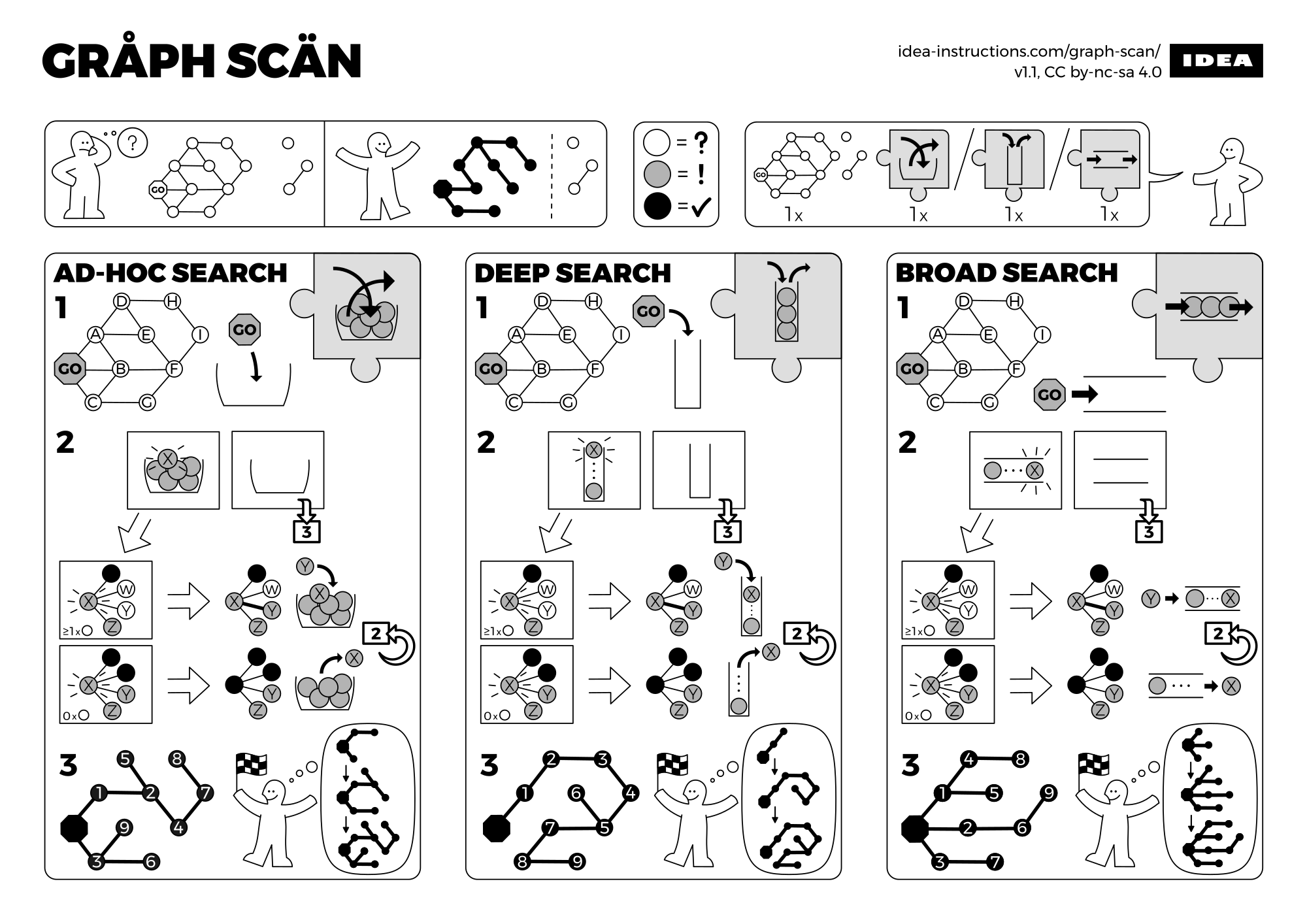# Graph Search and Traversal Algorithms

## Introduction

Graph is a common data structure that represents the relationships between different entities using edges and vertices. In practice, a graph can be extremely large, and there is a need to traverse or search the graph along the edges. Breadth-first search and depth-first search algorithms are the most commonly used traverse and search algorithm for graphs.In this blog post, I would like to discuss the implementations of directed and undirected graphs, breath-first and depth-first search algorithms.

## Graph Search and Traversal

Graph search and traversal are fundamentally the same. We will use the terms, search and traversal, interchangeably.

### Graph Representation and Implementation

Graph is commonly represented using adjacency matrix or adjacency list. Adjacency matrix is not favored for large graphs because usually the edges in large graphs are sparse. Adjacency list uses the least amount of the memory, but querying the specified neighbor of a node can be inefficient in a graph whose edges are dense. So we uses adjacency set instead which is fast for query and not too bad for memory.

Graph can be directed and undirected. There could also be isolated vertices in the graph that have no edges. There could also be vertices that connects with themselves. These graph features should not be ignored and have to be implemented accordingly.

The breadth-first search algorithm explores all the nodes at the current level before it explores the next level. To achieve this, it uses a first-in-first-out (FIFO) queue.

### Depth-First Search (DFS)

The depth-first search algorithm explores as far as possible along each branch before backtracking. It can be implemented recursively or iteratively. The recursive algorithm naturally explores the depth first but is not favored for large graphs because of stack overflow. The iterative algorithm uses a last-in-first-out (LIFO) stack to explore the depth first.

### Example

We created a dummy directed graph and a dummy undirected graph to test our search algorithms.

The expected outputs from the terminal are

Note that the iterative and recursive depth-first search algorithms do not necessarily result in the same traversal order.

### Source Code

All the source code could be found on GitHub Gist.

## Miscellaneous

Many search and traversal applications in practice are implemented using modified breath-first and depth-first search algorithms.

Lei Mao

07-13-2021

07-13-2021